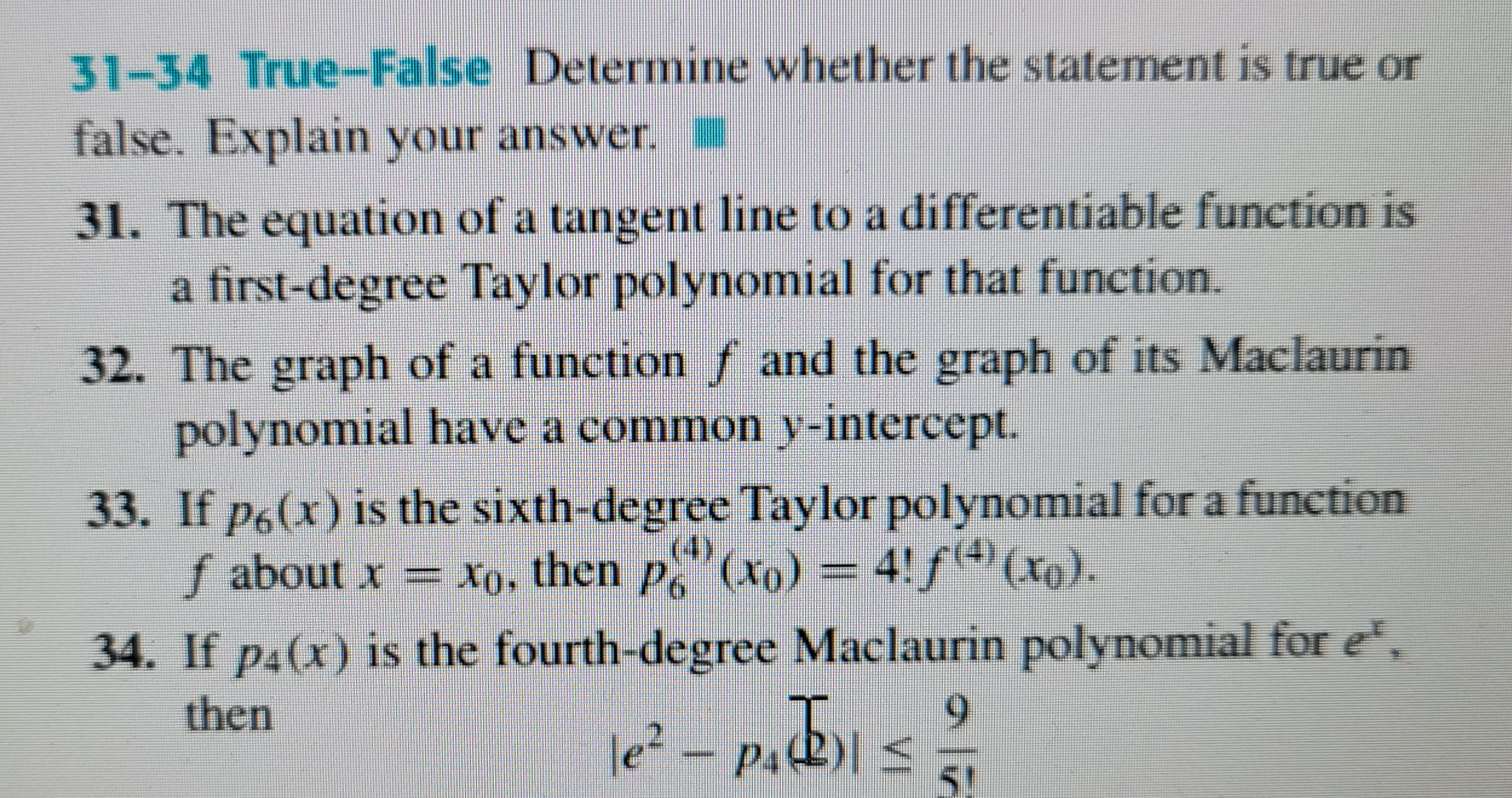### ¿Todavía tienes preguntas de matemáticas?

Pregunte a nuestros tutores expertos
Algebra
Pregunta31-34 True-False Determine whether the statement is true or false. Explain your answer. 31. The equation of a tangent line to a differentiable function is a first-degree Taylor polynomial for that function. 32. The graph of a function $$f$$ and the graph of its Maclaurin polynomial have a common $$y$$ -intercept. 33. If $$p _ { 6 } ( x )$$ is the sixth-degree Taylor polynomial for a function

$$f$$ about $$x = x _ { 0 }$$ , then $$p _ { 6 } ^ { ( 4 ) } ( x _ { 0 } ) = 4 ! f ^ { ( 4 ) } ( x _ { 0 } )$$ . 34. If $$p _ { 4 } ( x )$$ is the fourth-degree Maclaurin polynomial for $$e ^ { x }$$ , then

$$e ^ { 2 } - p _ { 4 } ( \overline { 2 } ) | \leq \frac { 9 } { 5 ! }$$

$$|e^{2}- p_{4}(2)|= R_{4}(2)= \frac{e^{\varepsilon }}{5!}2^{5},\varepsilon \in(0,2) \\\Rightarrow |e^{2}- p_{4}(2)|> \frac{e^{0}}{5!}2^{5}= \frac{32}{5!}> \frac{9}{5!}$$